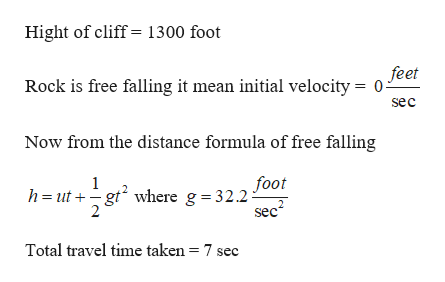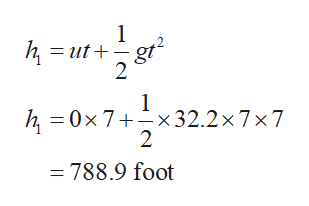# Suppose a rocks falls vertically off of a 1300 foot cliff. Use the distance equation for an object in free fall, , to determine the rock's distance from the ground, in feet, after 7 seconds of free fall. (Enter an exact number as an integer, fraction, or decimal.)  ft

Question
2 views

Suppose a rocks falls vertically off of a 1300 foot cliff. Use the distance equation for an object in free fall, , to determine the rock's distance from the ground, in feet, after 7 seconds of free fall. (Enter an exact number as an integer, fraction, or decimal.)
ft

check_circle

Step 1

Consider the given informationhelp_outlineImage TranscriptioncloseHight of cliff= 1300 foot feet Rock is free falling it mean initial velocity = sec Now from the distance formula of free falling foot h ut gt where g = 32.2- sec 1 Total travel time taken = 7 sec fullscreen
Step 2

Now substitute these all value in giv...help_outlineImage Transcriptionclose1 h, ut gt 2 1 h, 0x 7+x 32.2 x 7 x 7 2 788.9 foot fullscreen

### Want to see the full answer?

See Solution

#### Want to see this answer and more?

Solutions are written by subject experts who are available 24/7. Questions are typically answered within 1 hour.*

See Solution
*Response times may vary by subject and question.
Tagged in

### Other